# BIT110 Business Mathematics and Statistics Oz Assignments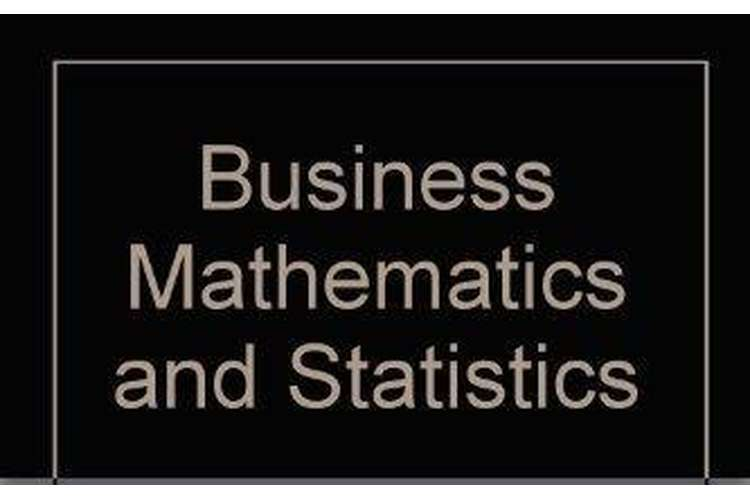## Introduction

Business mathematics and statistics contains the details about the salary of bachelor degree graduates in Australia. This reporteddeal with the 23 different bachelor courses. Into this reports all the analysis of the salary of the different courses. The data deals with the year from 1999 to 2015. After analysis, all the details of the different courses over the 16 years, forecast the salary of the years from 2016 to 2025. There are two different analysis perform, one is linear regression and other is the moving average business analysis. There is some variation in the values from both the analysis. Into the report, there will be using the moving average, for calculating the average salary of the year 2016 to 2025. In the calculation for the year 2016,it will take the average of the years from 2015 to 2012. Same calculation for the year 2017 to 2019, the average values of the salary changes. For the year 2017, it will be from 2016 to 2013. For the year2018, it will be 2017 to 2014 and for the year 2019, it will be 2018 to 2015. After that for the year of 2020, it will be taking anaverage of five years from 2015 to 2019. Same process for the other years from 2021 to 2024. In the end for the year 2025, it will take an average of 10 years from 2015 to 2024.

### Purpose of the report

This assignment provides the details of the salary of the various bachelor courses. This report mainly deals with the forecasting values of salary for the years 2016 to 2025. For calculating the values of there are two different analysis will be performed. Other than this, thereport provides the information about the how the calculation is preforms and the working of the two different methods. Into report, different graphsrelated to the different salaries of the bachelor’sdegreesgraduate over the spam of the year 2016 to 2025 based on the years 1999 to 2015. All the data for the calculation and finding the values of the salary are taken from the graduatecareers website (Careers, n.d.).

### Findings

Into the report, there are finding over the salaries of the different years. With the use of the previous years. Analysis of the findings. Forecasting of the salaryis to complete with the use of two different approaches one is by the use of moving average analysis and other is based on the linear regression analysis. The below graph shows the values of the salary of 2016 with the use of moving average analysis.

Calculation part with the use of moving average analysis.For the calculation of the year 2016, taken the values of the year 2012 to 2015, four-year spam.In addition, the same calculation for the year 2017 to 2019. Moreover, for the calculation of the year 2020, will be calculation is done by taking the values from 2015 to 2019, five years.In addition, same calculation into the years 2021 to 2024. Moreover, for the calculation of the year 2025, taking the values for the year 2015 to 2024, 10 years (Nelson, 2017).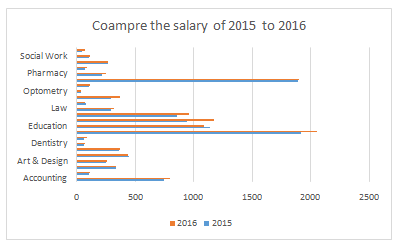After that, one analysis will be done with the use of the forecasted values of the year 2016, 2017 and 2025. Calculation part is complete with the use of moving average analysis (Excel, n.d.).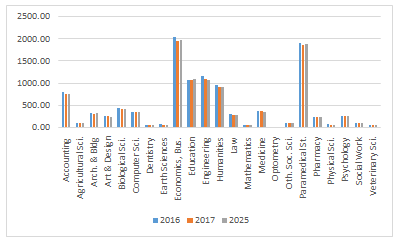After that, one analysis is done on the calculation of2024 to 2025. Because in this part of the calculation, there is average year taken off 10 years.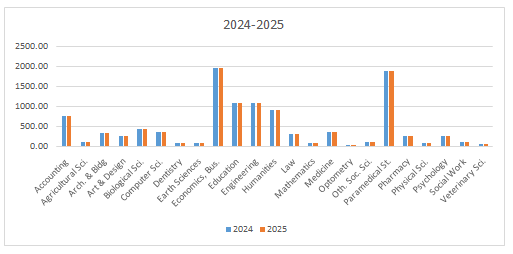These all are the part of the calculation done with the use of the moving average analysis. Below shows that the analysis based on the linear regression.

The first analysis is done over the year of 2015 and 2016.  In the calculation, there is taking the values form the regression analysis. The values of the depended variable and r will be foundbased on the salary in 2015. The values of the independent variable are21.63819435 and value of r will be 0.984835931. With the use of the calculation, there are different calculation performed to calculate the salary of the years 2016 with the use of salary of 2015. Below shows the graphs of the changes, that comes into the values of the salary.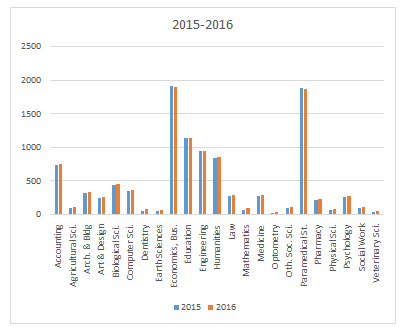After that other calculation is done with the use of the all the forecasted values of the years 2016, 2017 and 2025. With the use of linear regression (Zaiontz, 2017).

### Conclusion

Business mathematics and statistics, there are different analysis, performed and calculation for the analysis is also cover into this report. This reportstarts with the summary of the report and end with the calculation part of the analysis.Calculation and analysis part of the reports starts with the use of themethod, business strategy, moving average analysis.Calculate the forecasted values of the years 2016 to 2025. With the use of different approaches. For the years, 2016 to 2019 there is anaverageof the last four years. After that for the year of 2020. For this year taken the average of last five years. Last for the year of 2025 taken the average of thelast 10 years. For the calculation there are different graphs are attachedto the report. After that next part starts with the calculation with the use of linear regression. Into this whole calculation is based on the depended values,in-depended values and r. r is thecoefficient of regression.All the values calculated into the report and, with the use of the values forecasted salary of the years from 2016 to 2025 are also calculated into the report.

### References

1. Careers, G. (n.d.). GradStats - Graduate Careers Australia. [online] Graduate Careers Australia. Available at: http://www.graduatecareers.com.au/research/researchreports/gradstats/ [Accessed 7 Nov. 2017].
2. Excel (n.d.). Moving Average in Excel. [online] Excel-easy.com. Available at: http://www.excel-easy.com/examples/moving-average.html [Accessed 7 Nov. 2017].
3.
LARSON, B. (2016). Linear Regression using Excel. [online] Analytics4All. Available at: https://analytics4all.org/2016/03/31/linear-regression-using-excel/ [Accessed 7 Nov. 2017].
4.
Nelson, S. (2017). How to Calculate Moving Averages in Excel - dummies. [online] dummies. Available at: http://www.dummies.com/software/microsoft-office/excel/how-to-calculate-moving-averages-in-excel/ [Accessed 7 Nov. 2017].
5.
Zaiontz, C. (2017). Regression Analysis | Real Statistics Using Excel. [online] Real-statistics.com. Available at: http://www.real-statistics.com/regression/regression-analysis/ [Accessed 7 Nov. 2017].